Ordinary differential equations (ODEs)¶

Initial value problems¶

Recall the logistic equation for a population $N$ at time $t$: $$\dot{N} = r \, N\, \bigg(1 - \dfrac{N}{K}\bigg)$$ where $r$ is the rate of growth, $K$ is the carrying capacity.

In :
%matplotlib inline

In :
import numpy as np
import matplotlib.pyplot as plt

In :
from scipy.integrate import odeint

def f(N, t, r, K):
return r * N * (1 - N/K)

r = 1.
K = 50.
N0 = 10.
t = np.linspace(0., 10, 100)

N = odeint(f, N0, t, args=(r,K))
plt.plot(t, N)
plt.ylim([0, 100])
plt.axhline(y=K, color='k', linewidth=0.5)
plt.show()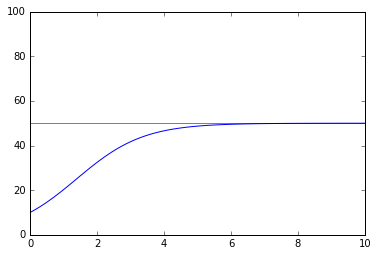In :
N0 = 80
t = np.linspace(0., 10, 100)

N = odeint(f, N0, t, args=(r,K))
plt.plot(t, N)
plt.ylim([0, 100])
plt.axhline(y=K, color='k', linewidth=0.5)
plt.show()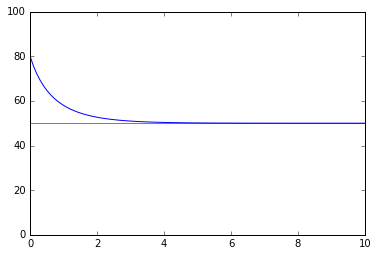In :
N0 = 30
t = np.linspace(0., 10, 100)

N = odeint(f, N0, t, args=(r,K))
plt.plot(t, N)
plt.ylim([0, 100])
plt.axhline(y=K, color='k', linewidth=0.5)
plt.show()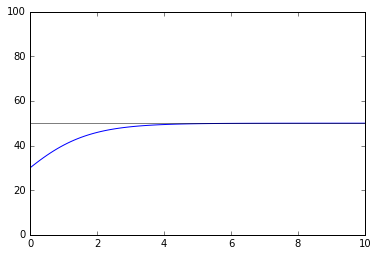Here is another example.

In :
%matplotlib inline
import numpy as np
import matplotlib.pyplot as plt
from scipy.integrate import odeint

def f(x, t):
return x * (1 - x)

t = np.linspace(0, 5, 100) # <- time slices to compute
x0 = 0.1                   # <- initial condition
x = odeint(f, x0, t)       # <- solve ODE

plt.figure()
plt.plot(t, x)
plt.show()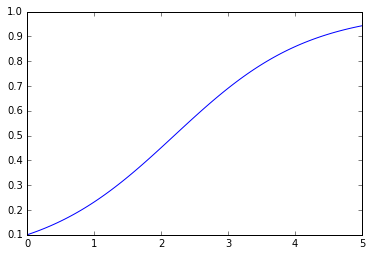Higher order equations, or systems of first order equations¶

odeint accepts only first order systems. Since higher order equations (those with higher than first derivatives) can be written as a system of first order equations, this is not a real limitation.

Here is an example of solving a system of first order ODEs.

In :
%matplotlib inline
import numpy as np
import matplotlib.pyplot as plt
from scipy.integrate import odeint

def f(state, t):
x, y = state           # <- now odeint gives us an array of data
return np.array([      # <- we'll return an array representing the vector field at the point
-y,
x,
])

t = np.linspace(0, 8, 100) # <- time slices to compute
x0 = np.array([1.0, 1.0])  # <- initial condition (now an array!)
soln = odeint(f, x0, t)    # <- solve ODE

plt.figure()
plt.plot(soln[:, 0], soln[:, 1])
plt.show()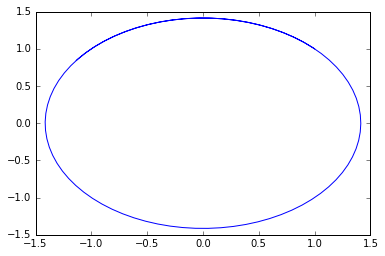Plotting 2D vector fields¶

To visualize a 2D vector field on plane we can use quiver or streamplot from Matplotlib.

In :
%matplotlib inline
import numpy as np
import matplotlib.pyplot as plt

x, y = np.meshgrid(np.linspace(-1, 1, 25),
np.linspace(-1, 1, 25))

u = -y
v = x

plt.figure(figsize=(8, 8))
plt.xlim(-1, 1)
plt.ylim(-1, 1)
plt.quiver(x, y, u, v)
plt.show()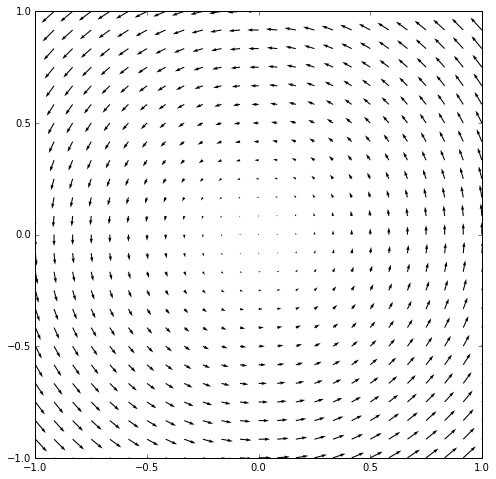In :
plt.figure(figsize=(8, 8))
plt.xlim(-1, 1)
plt.ylim(-1, 1)
plt.streamplot(x, y, u, v)
plt.show()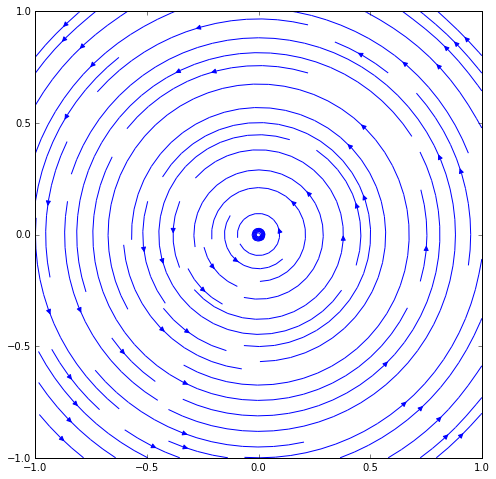The stream plot gives a better view of the flow of the vector field but the magnitudes are not visible. They can be brought in by coloring the streamlines based on the magnitude of the vector field.

In :
from numpy.linalg import norm

# dstack takes our 2D arrays of u, v values and combines
# them into a coherent 2D array of (u, v) pairs so the norm
# for each vector can be computed easily.
mag = norm(np.dstack([u, v]), axis=-1)
print np.dstack([u, v]).shape
print u.shape
plt.figure(figsize=(10, 8))
plt.xlim(-1, 1)
plt.ylim(-1, 1)
plt.streamplot(x, y, u, v, color=mag, cmap='autumn')
plt.colorbar()
plt.show()

(25, 25, 2)
(25, 25)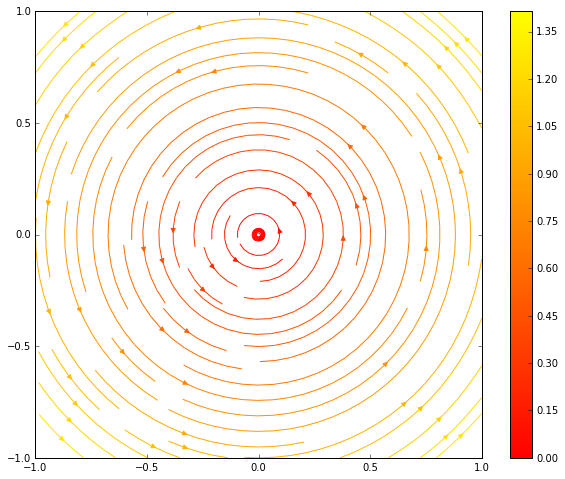Boundary value problems for ODEs¶

To be continued ...

Partial differential equation (PDEs)¶

Introduction to the various methods and tools and where the software for each is available.

Hyperbolic PDEs¶

Here we will only work with the most basic linear hyperbolic PDE : the linear advection equation $u_t + c u_x = 0$ on $\mathbb{R}$ with initial value $u_0:\mathbb{R}\rightarrow \mathbb{R}$. For any serious work on hyperbolic PDEs, you should consider using a good package. The package CLAWPACK now has a Python interface called PyCLAW. See the gallery of PyCLAW results for examples of its power and convenience.

The examples below are given to illustrate the difficulties that can arise in even the simplest linear hyperbolic PDEs. The difficulties here are nothing compared to the difficulties such as development of shocks in nonlinear PDEs such as Burgers' equation.

Consider linear advection with wave moving to the right with speed $c = 1$. The seemingly obvious method of using forward in time space discretization of the derivatives does not work.

Define and plot the initial condition:

In :
from numpy import linspace, zeros
import matplotlib.pyplot as plt

def u0(x):
return (1 - abs(x)) * (abs(x) < 1)

x_fine = linspace(-2, 3, 201, endpoint=True)
plt.figure()
plt.plot(x_fine, u0(x_fine), '0.5')
plt.show()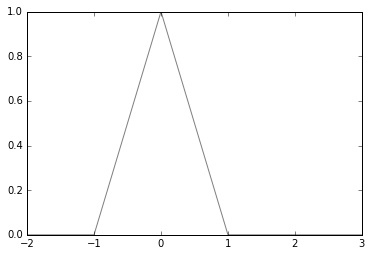Set the parameters:

In :
c = 1.; final_time = 0.8
M = 20
h = 5./(M+1) # spatial discretization parameter
r = 0.8 # ratio of spatial and time discretization parameter
k = h*r # time discretization parameter


Now implement the forward in time, forward in space scheme:

In :
from math import floor

plt.plot(x_fine, u0(x_fine), '0.5')
num_timesteps = int(floor(final_time/k))
x = linspace(-2, 3, M+2)
u = zeros((2, M+2))
now = 0; new = 1
u[now] = u0(x)
for i in range(1, num_timesteps+1):
u[new][1:M+1] = (1+c*r)*u[now][1:M+1] - c*r*u[now][2:M+2]
u[new, M+1] = u[new, M]
now = new; new = int(not new)
plt.plot(x, u[now], 'o-')
plt.title('FTFS ' + 'time = %2.4f,  ' %  (k*num_timesteps) + 'k/h = %g' % r)
plt.show()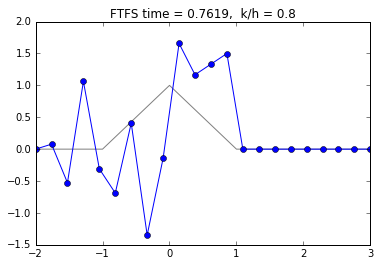Does not seem to work. However, the forward in time and backward in space does work:

In :
plt.figure()
plt.plot(x_fine, u0(x_fine), '0.5')
now = 0; new = 1
u[now] = u0(x)
for i in range(1, num_timesteps+1):
u[new][1:M+1] = c*r*u[now][0:M] + (1-c*r)*u[now][1:M+1]
u[new, M+1] = u[new, M]
now = new; new = int(not new)
plt.plot(x, u[now], 'o-')
plt.title('FTBS ' + 'time = %2.4f,  ' %  (k*num_timesteps) + 'k/h = %g' % r)
plt.show()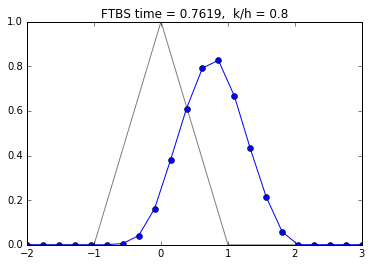The choice of $k/h$ is important. Let's try a value of $k/h > 1$:

In :
r = 1.2
k = h*r
num_timesteps = int(floor(final_time/k))
plt.figure()
plt.plot(x_fine, u0(x_fine), '0.5')
now = 0; new = 1
u[now] = u0(x)
for i in range(1, num_timesteps+1):
u[new][1:M+1] = c*r*u[now][0:M] + (1-c*r)*u[now][1:M+1]
u[new, M+1] = u[new, M]
now = new; new = int(not new)
plt.plot(x, u[now], 'o-')
plt.title('FTBS ' + 'time = %2.4f,  ' %  (k*num_timesteps) + 'k/h = %g' % r)
plt.show()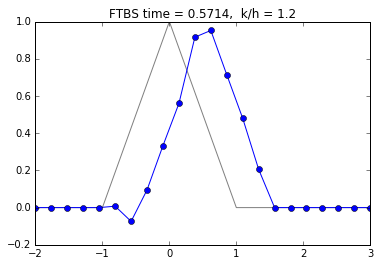Let's try for longer time:

In :
final_time = 1.5
num_timesteps = int(floor(final_time/k))
plt.figure()
plt.plot(x_fine, u0(x_fine), '0.5')
now = 0; new = 1
u[now] = u0(x)
for i in range(1, num_timesteps+1):
u[new][1:M+1] = c*r*u[now][0:M] + (1-c*r)*u[now][1:M+1]
u[new, M+1] = u[new, M]
now = new; new = int(not new)
plt.plot(x, u[now], 'o-')
plt.title('FTBS ' + 'time = %2.4f,  ' %  (k*num_timesteps) + 'k/h = %g' % r)
plt.show()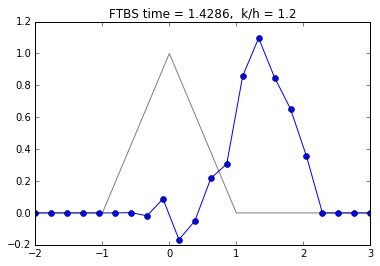The instability is now obvious.

A more interesting classical scheme is the Lax-Friedrichs scheme:

In :
final_time = 0.8
r = 0.8
k = h*r
num_timesteps = int(floor(final_time/k))
plt.figure()
plt.plot(x_fine, u0(x_fine), '0.5')
now = 0; new = 1
u[now] = u0(x)
for i in range(1, num_timesteps+1):
u[new][1:M+1] = (1+c*r)/2*u[now][0:M] + (1-c*r)/2*u[now][2:M+2]
u[new, M+1] = u[new, M]
now = new; new = int(not new)
plt.plot(x, u[now], 'o-')
plt.title('Lax-Friedrichs ' + 'time = %2.4f,  ' %  (k*num_timesteps) + 'k/h = %g' % r)
plt.show()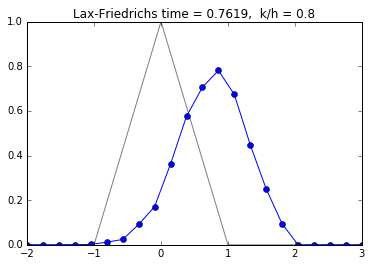Elliptic PDEs¶

Poisson equation is used as a model linear elliptic PDE and is numerically solved using finite difference or finite element methods. A modern Python library for finite elements is FEniCS. We can see some example programs here. When solving PDEs one often needs other tools to create a triangulation of the domain and to visualize the results. For visualization you may consider Paraview, a powerful data visualizer. For triangulating 2D planar domains Triangle is a powerful and standard free tool. There are also some good 3D tetrahedralization tools such as tetgen.